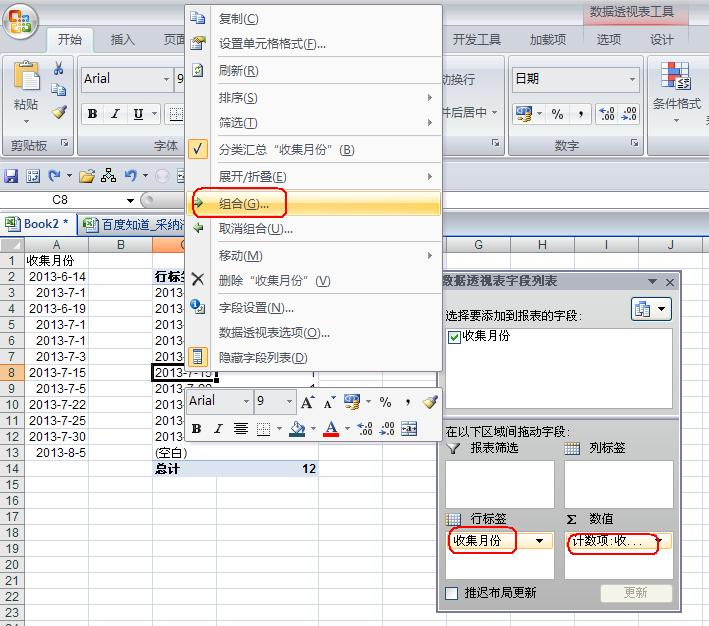• ZOL首页
• 产品报价
• 论坛
• ZOL问答
• 更多
• 手机客户端

Excel中怎么统计某个数出现的次数?

Excel中怎么统计某个数出现的次数?

• 回答数

7

• 浏览数

9,106

### 7个回答 默认排序 默认排序 按时间排序

=countif(A:A,某数)

=countif(A1:D100,某数)

=SUMPRODUCT((A\$2:A\$1001=F2)*(B\$2:B\$1001>=H\$2)*(B\$2:B\$1001<=H\$3))

=COUNTIFS(A:A,F2,B:B,">="&H\$2,B:B,"<="&H\$3)=sumproduct((f3:f1000<>"")*(month(f3:f1000)=1)),这样子

### 最新回答 更多>

ZOL问答 > Excel中怎么统计某个数出现的次数?

### 举报成功